DG7YBN / 14 MHz / YBN 14-5w rmc   Last Update Oct 24th, 2020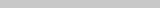.......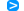Performance Data and GeometryPattern and VSWRPattern and VSWR over GroundValidation of Tapering YBN 14-5w rmc   50 ohms Yagi with Conventional Driven Element The YBN 14-5w full size boom length is a 50 ohms wideband beam covering the full 20 m band. Design date of issue: 2020.10.24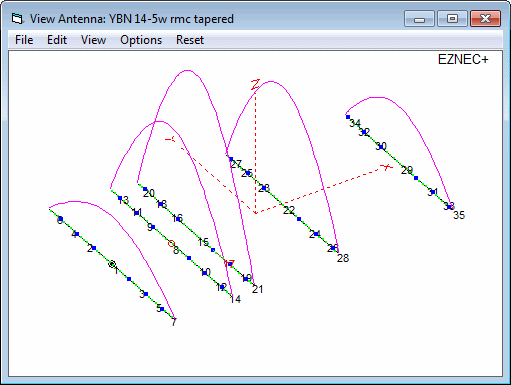Free space 3D pattern (14.3 MHz)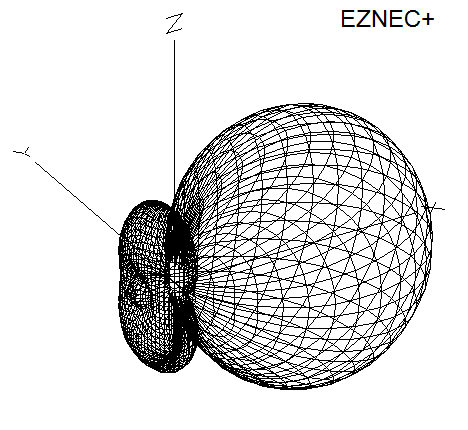Performance Data Specs: with tapered elements (30 > 25 > 20 > 16 mm) free space ``` 14.2 MHz 14.3 MHz Gain vs. isotr. Rad. 10.03 dBi 10.20 dBi Gain vs. Dipole 7.88 dBD 8.05 dBD -3 dB E-plane 55.4 deg. -3 dB H-plane 74.4 deg. F/B -28.9 dB F/R -19.2 dB Impedance 50 ohms VSWR Bandwidth 14.0 MHz = 1:1.06, 14.35 = 1:1.05 Mechan. Length 15.44 m exclusive boom offsets for elem. plates Electr. Length 0.73 λ (14.2 MHz) vert .Stacking Dist. 17.54 m or 57.5 ft ``` How many HF operators have been looking up this design since Oct. 2020?Geometry ... is available in request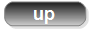Pattern and VSWR Plots Elevation and Azimuth plot at 14.3 MHzRL and SWR plots - simulated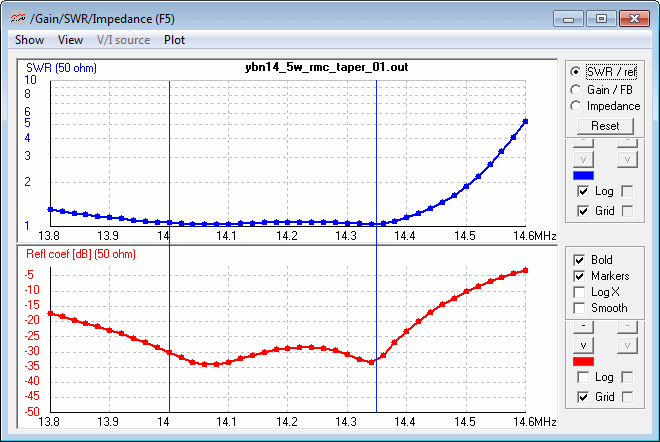Tapered Model Azimuth plot 14.1 - 14.35 MHz14.10 MHz    F/B = 21.7 dB    Gain =   9.86 dBi 14.20 MHz    F/B = 28.9 dB    Gain = 10.03 dBi 14.30 MHz    F/B = 28.6 dB    Gain = 10.20 dBi 14.35 MHz    F/B = 25.2 dB    Gain = 10.28 dBiPattern and VSWR Plots over Ground • At height of 16 m (perfect gnd assumed) Azimuth plot at 14.1 MHz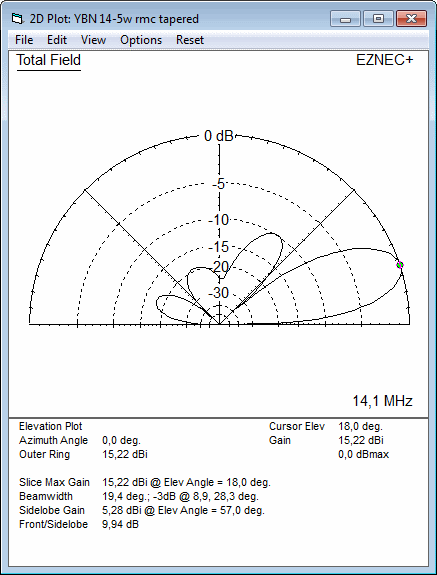Azimuth plot at 14.3 MHzRL and SWR plots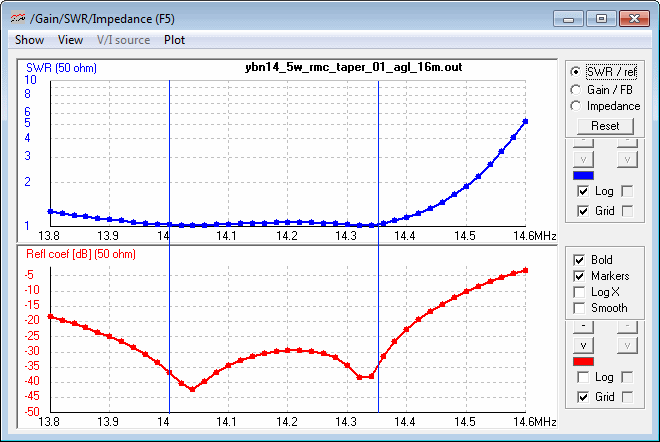• At height of 24 m (perfect gnd assumed) Azimuth plot at 14.1 MHz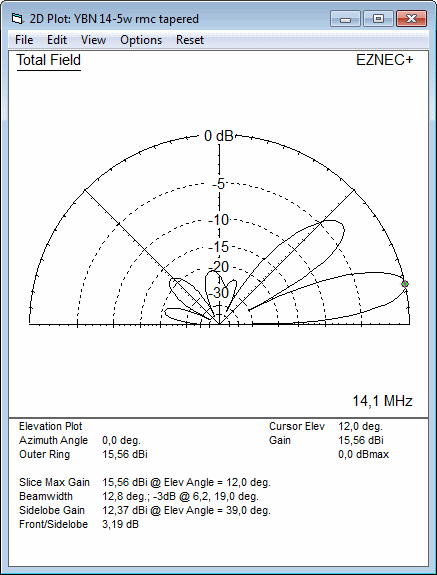Azimuth plot at 14.3 MHz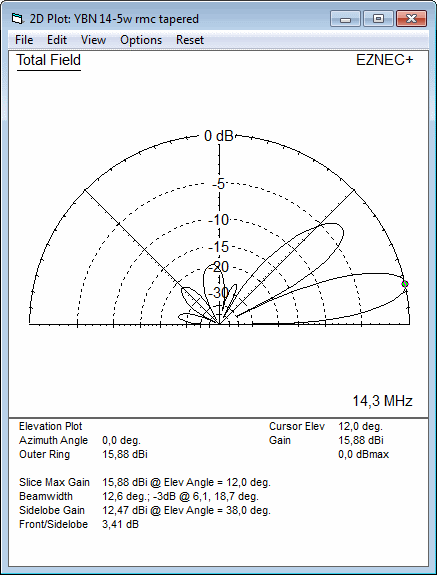RL and SWR plots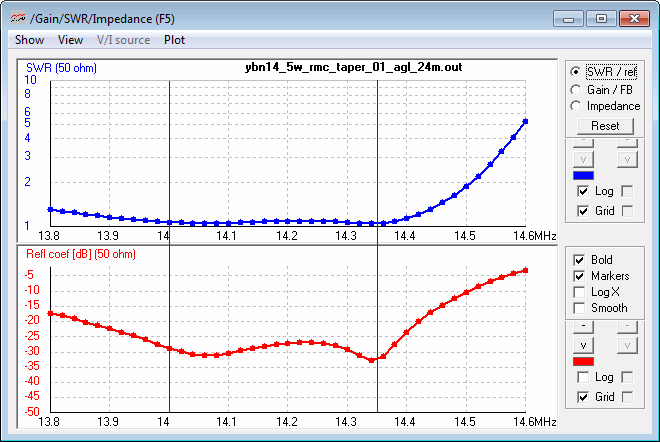Validation of Tapering Tapered Yagi: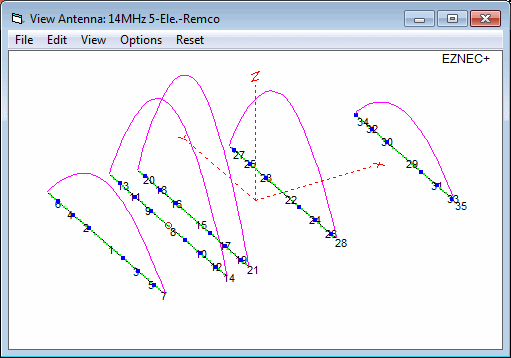Setting 4nec2 for the task (Stepped radius correction)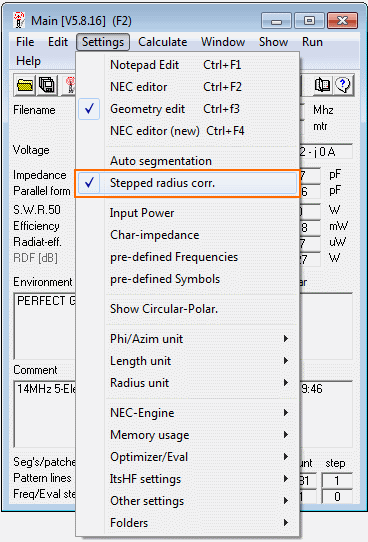Average Gain with zero wire losses = 1.000 = 0.00 dB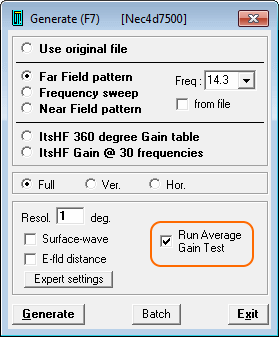Average Gain with Aluminium T-6061 for Elements = 0.993 = -0.03 dB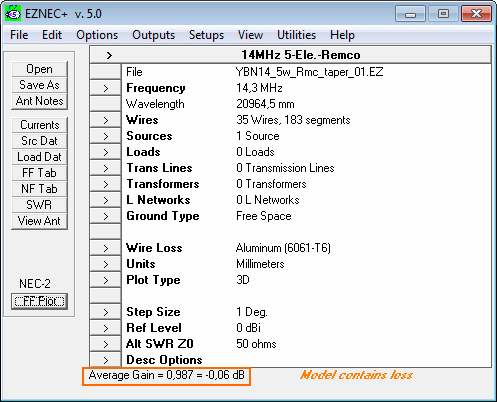73, Hartmut, DG7YBN